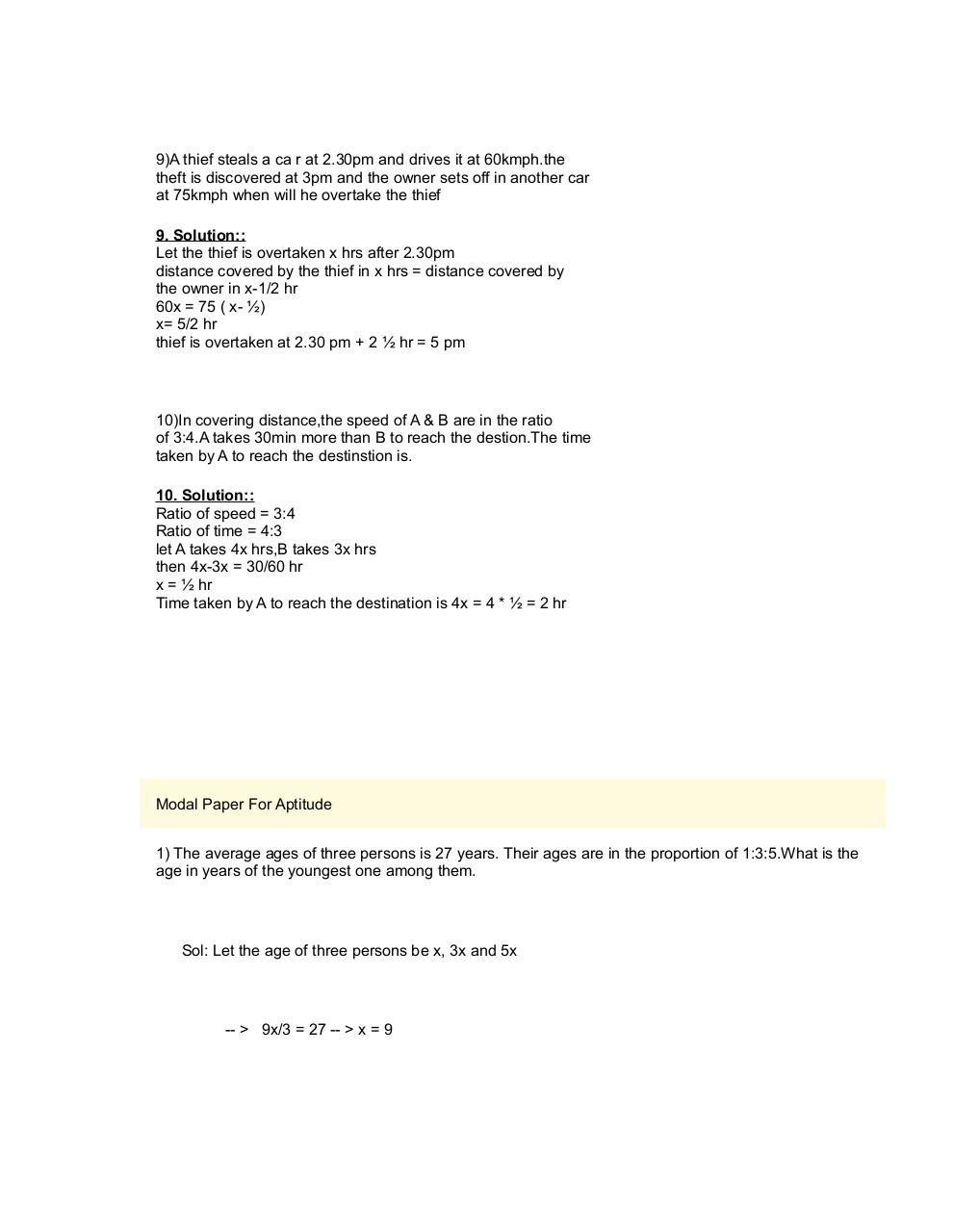# PDF Archive

Easily share your PDF documents with your contacts, on the Web and Social Networks.Page 1 2 34572

#### Text preview

9)A thief steals a ca r at 2.30pm and drives it at 60kmph.the
theft is discovered at 3pm and the owner sets off in another car
at 75kmph when will he overtake the thief
9. Solution::
Let the thief is overtaken x hrs after 2.30pm
distance covered by the thief in x hrs = distance covered by
the owner in x-1/2 hr
60x = 75 ( x- ½)
x= 5/2 hr
thief is overtaken at 2.30 pm + 2 ½ hr = 5 pm

10)In covering distance,the speed of A &amp; B are in the ratio
of 3:4.A takes 30min more than B to reach the destion.The time
taken by A to reach the destinstion is.
10. Solution::
Ratio of speed = 3:4
Ratio of time = 4:3
let A takes 4x hrs,B takes 3x hrs
then 4x-3x = 30/60 hr
x = ½ hr
Time taken by A to reach the destination is 4x = 4 * ½ = 2 hr

Modal Paper For Aptitude
1) The average ages of three persons is 27 years. Their ages are in the proportion of 1:3:5.What is the
age in years of the youngest one among them.

Sol: Let the age of three persons be x, 3x and 5x

-- &gt; 9x/3 = 27 -- &gt; x = 9